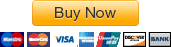## Dynamics ENGR 212-Ecampus| Oregon State University| Assignment #1 Ver. A

###### You can buy the complete solutions to the problems that follow. You will be directed to the solution file as soon as you will complete payment. Please do not close or refresh browser until then. If something went wrong, please send an email to enggmathem@gmail.com.Problem #1:

Problem #1, Part a:
Determine the time (in seconds) required for a train to travel 1 [km] along its rails if the train starts from rest, reaches a maximum speed at some intermediate point, and then stops at the end of the road. The train can accelerate at 1.5 [m/(sˆ2)] and decelerate at 2 [m/(sˆ2)].

Problem #1, Part b:
The acceleration of a asteroid traveling a straight line is a=(1/4)s^(1/2)[m/(sˆ2)], where s is in meters. If v = 0 [m/s], s= 1 [m] when t = 0 seconds, determine the particle’s velocity (in [m/s]) at s = 2 [m].

Problem #2:

Problem #2 Part a:
A train travels along a straight route with an acceleration-deceleration described by Figure1. If the train starts from rest, determine the distance, s’, the car travels until it stops. Construct the v–s graph (in terms of ft/s and feet) for 0≤s≤s’. Construct the graph by hand, but be sure to label it appropriately.

Problem #2 Part b:
A man riding upward in a freight elevator accidentally drops a package oﬀ the elevator when it is 100 feet from the ground. If the elevator maintains a constant upward speed of 4 [ft/s], determine how high (in feet) the elevator is from the ground the instant the package hits the ground. Draw the v–t curve (in terms of ft/s and seconds) for the package during the time it is in motion. Assume that the package was released with the same upward speed as the elevator.

Problem #3

Problem #3 Part a:
The van travels over the hill described by$y=\left(-1.5\left(10^{-3}\right)x^2+15\right)$ [ft]; see Figure2. If it has a constant speed of 75 [ft/s], determine the x and y components of the van’s velocity and acceleration when x = 50 [ft].Problem #3 Part b:
The motorcycle travels with constant speed$v_0$ along the path that, for a short distance, takes the form of a sine curve; see Figure3. Determine the x and y components of its velocity at any instant on the curve.Problem #4:
A projectile is given a velocity$v_0$; see Figure4. Determine the angle,$\phi$ (in radians), at which it should be launched so that, d, is a maximum. The acceleration due to gravity is g.

Problem #5:
The baseball player A hits the baseball at$v_A=40\:\left[ft/s\right]$ and$\theta _A=60^{\circ}$ from the horizontal. When the ball is directly overhead of player B he begins to run under it; see Figure5. Determine the constant speed (in [ft/s]) at which B must run and the distance d (in feet) in order to make the catch at the same elevation at which that ball was hit.